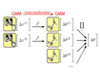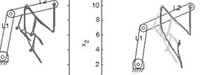### Abstract

We present a probabilistic architecture for solving generically the problem of extracting the task constraints through a Programming by Demonstration (PbD) framework and for generalizing the acquired knowledge to various situations. We propose an approach based on Gaussian Mixture Regression (GMR) to find automatically a controller for the robot reproducing the essential characteristics of the skill by handling simultaneously constraints in joint space and in task space. Experiments with two 5-DOFs Katana robots are then presented with two manipulation tasks consisting of handling and displacing a set of objects.

### Bibtex reference

@inproceedings{Calinon08IROS,
author="S. Calinon and A. Billard",
title="A Probabilistic Programming by Demonstration Framework Handling Skill Constraints in Joint Space and Task Space",
booktitle="Proc. {IEEE/RSJ} Intl Conf. on Intelligent Robots and Systems ({IROS})",
year="2008",
month="September",
location="Nice, France"
pages="367--372"
}

### Video

Incremental learning of a motion with two Katana robotic arms from Neuronics.
Two demonstrations are provided to show to the robots how to pour some liquid from a bottle into a glass. Then, the user moves manually one of the robot while the other robot follows as best as possible the learned motion in a flexible manner.

Video credit: Florent D'halluin.

### Source codesDownload Joint/task constraints sourcecode

#### Usage

Unzip the file and run 'demo1' in Matlab.

#### Reference

• Calinon, S. and Billard, A. (2008) A Probabilistic Programming by Demonstration Framework Handling Constraints in Joint Space and Task Space. IEEE/RSJ International Conference on Intelligent Robots and Systems (IROS), pp. 367-372.

#### Demo 1 - Demonstration of the use of Gaussian Mixture Regression (GMR) and inverse kinematics to reproduce a task by considering constraints both in joint space and in task spaceThis program shows the simulation of a robotic arm composed of 2 links and moving in 2D space. Several demonstrations of a skill are provided, by starting from different initial positions. The skill consists of moving each joint sequentially and then writing the alphabet letter 'N' at a specific position in the 2D space.
Constraints in joint space and in task space are represented through Gaussian Mixture Models (GMMs) and Gaussian Mixture Regression (GMR). By using an inverse kinematics process based on a pseudo-inverse Jacobian, the constraints in task space are then projected in joint space. By considering the projected constraints with the ones originally encoded in joint space, an optimal controller is found for the reproduction of the task. We see through this example that the system is able to generalize the learned skill to new robotic arms (different links lengths) and to new initial positions of the robot.